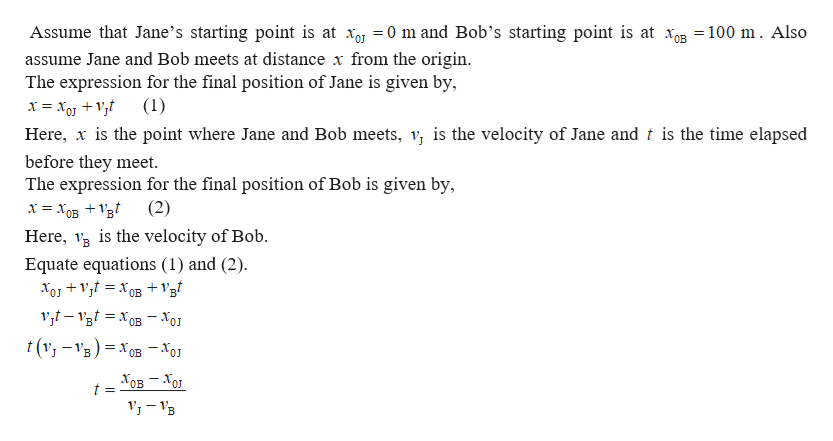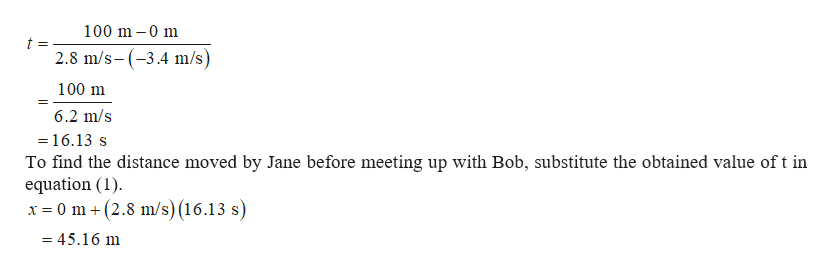# Jane and Bob see each other when 100 m apart. They are moving toward each other, Jane at speed 2.8m/s and Bob at speed 3.4m/s with respect to the ground. Find the time elapsed for Bob and Jane to meet up.Find the distance Jane moves before meeting up with Bob.

Question
13 views

Jane and Bob see each other when 100 m apart. They are moving toward each other, Jane at speed 2.8m/s and Bob at speed 3.4m/s with respect to the ground.

Find the time elapsed for Bob and Jane to meet up.

Find the distance Jane moves before meeting up with Bob.

check_circle

Step 1

Assume that the Jane and Bob are moving along the x-axishelp_outlineImage TranscriptioncloseAssume that Jane's starting point is at xoj = 0 m and Bob's starting point is at xB = 100 m. Also assume Jane and Bob meets at distance x from the origin The expression for the final position of Jane is given by, Here, is the point where Jane and Bob meets, v is the velocity of Jane and t is the time elapsed before they meet The expression for the final position of Bob is given by х %3D Хов + 1в Here, s the velocity of Bob (2) Equate equations (1) and (2) OB t(v-VB)x0B -xo = Хов — Хо OJ t = fullscreen
Step 2

Since Bob moves towards opposite to the positive x-direction, his velocity wi...help_outlineImage Transcriptionclose100 m-0 m t = 2.8 m/s-(3.4 m/s) 100 m 6.2 m/s =16.13 s To find the distance moved by Jane before meeting up with Bob, substitute the obtained value oft in equation (1) x 0 m(2.8 m/s) (16.13 s) = 45.16 m fullscreen

### Want to see the full answer?

See Solution

#### Want to see this answer and more?

Solutions are written by subject experts who are available 24/7. Questions are typically answered within 1 hour.*

See Solution
*Response times may vary by subject and question.
Tagged in

### Kinematics